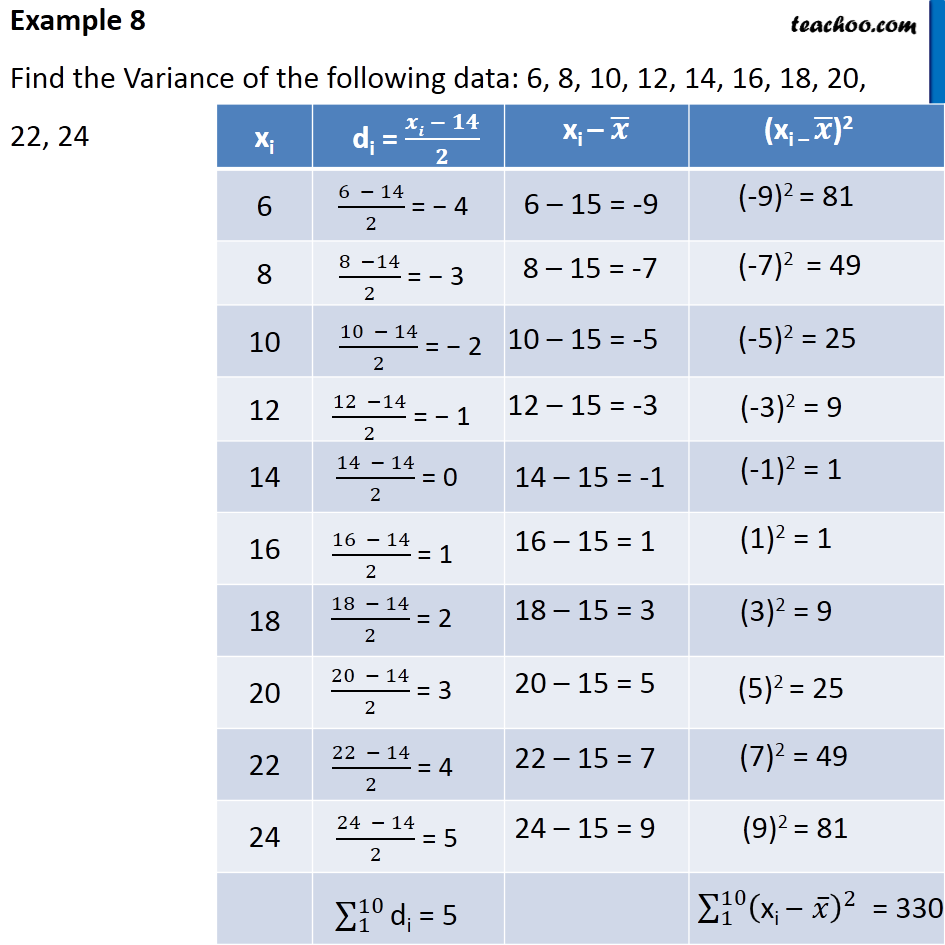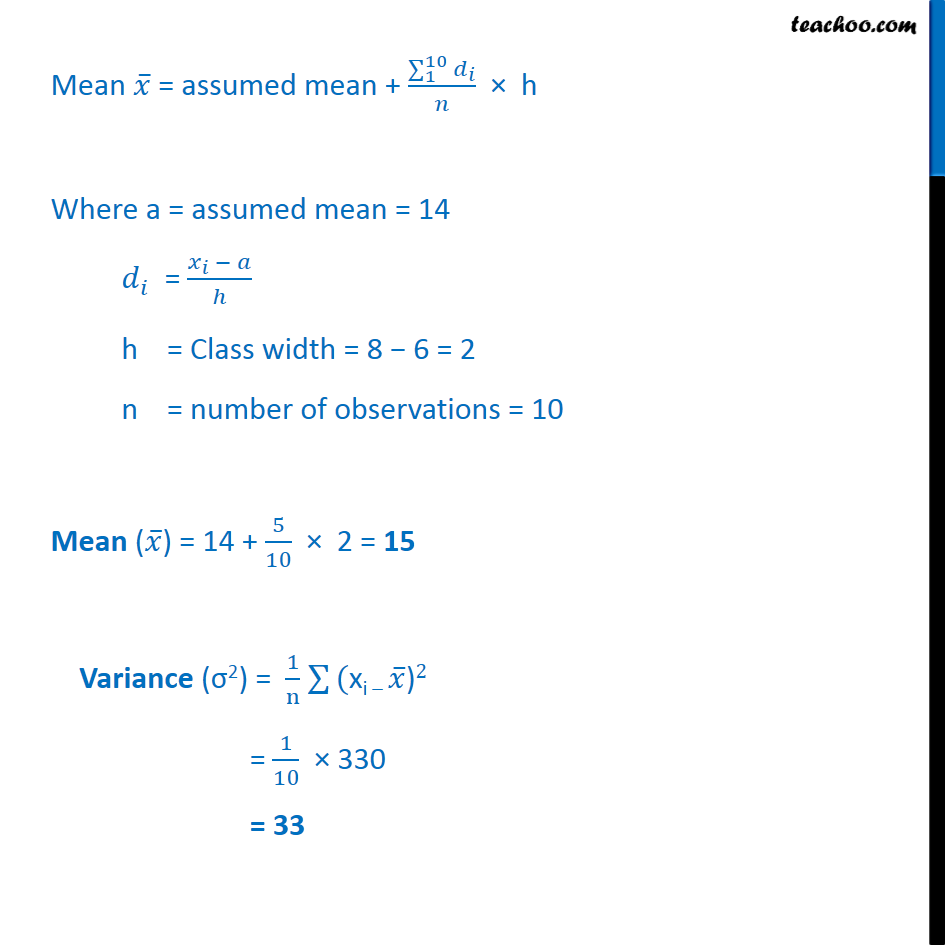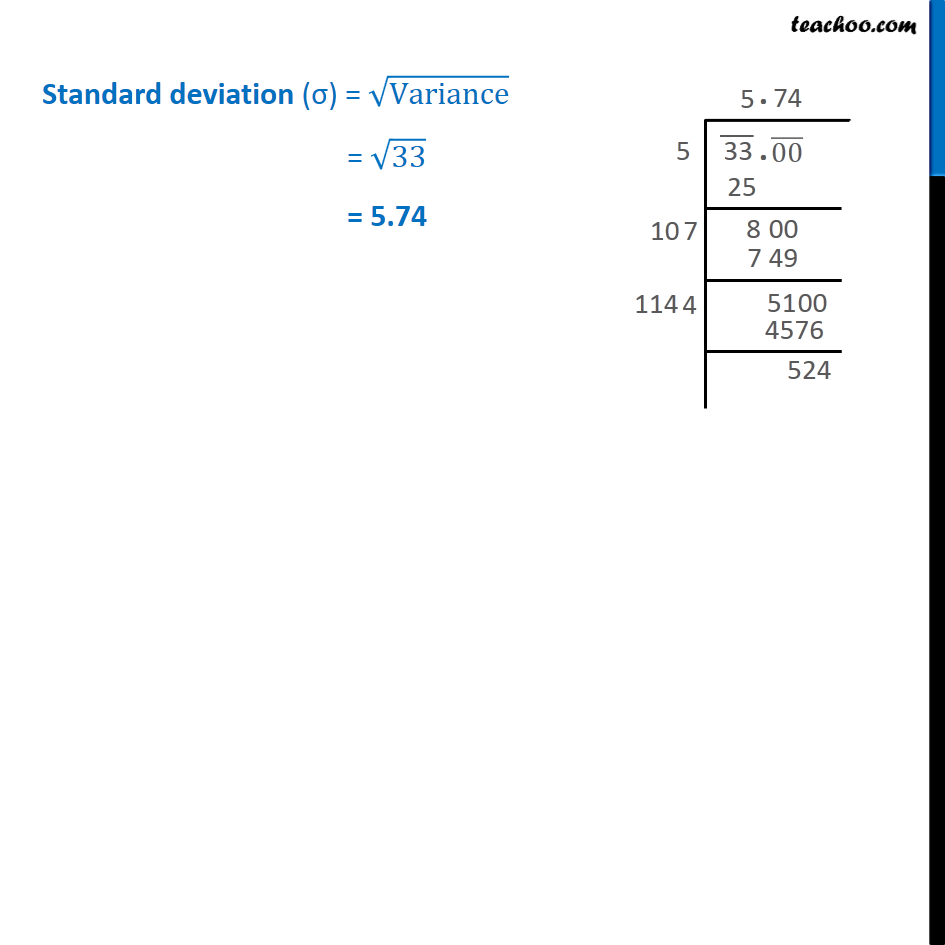Examples

Chapter 13 Class 11 Statistics
Serial order wiseLearn in your speed, with individual attention - Teachoo Maths 1-on-1 Class

### Transcript

Example 8 - Chapter 15 Class 11 Statistics - NCERT Find the Variance of the following data: 6, 8, 10, 12, 14, 16, 18, 20, 22, 24 xi di = (xi - 14)/2 xi – x ̅ ("xi – " x ̅)2 6 8 10 12 14 16 18 20 22 24 (6 − 14)/2 = − 4 (8 −14)/2 = − 3 (10 − 14)/2 = − 2 (12 −14)/2 = − 1 (14 − 14)/2 = 0 (16 − 14)/2 = 1 (18 − 14)/2 = 2 (20 − 14)/2 = 3 (22 − 14)/2 = 4 (24 − 14)/2 = 5 ∑_1^10▒"di" = 5 6 – 15 = -9 8 – 15 = -7 10 – 15 = -5 12 – 15 = -3 14 – 15 = -1 16 – 15 = 1 18 – 15 = 3 20 – 15 = 5 22 – 15 = 7 24 – 15 = 9 (-9)2 = 81 (-7)2 = 49 (-5)2 = 25 (-3)2 = 9 (-1)2 = 1 (1)2 = 1 (3)2 = 9 (5)2 = 25 (7)2 = 49 (9)2 = 81 ∑_1^10▒〖("xi – " 𝑥 ̅ )^2 〗 = 330 Mean 𝑥 ̅ = assumed mean + (∑_1^10▒𝑑_𝑖 )/𝑛 × h Where a = assumed mean = 14 𝑑_𝑖 = (𝑥_𝑖 − 𝑎)/ℎ h = Class width = 8 − 6 = 2 n = number of observations = 10 Mean (𝑥 ̅) = 14 + 5/10 × 2 = 15 Variance (σ2) = 1/n ∑▒〖"(xi – " 𝑥 ̅")" 2〗 = 1/10 × 330 = 33 Standard deviation (σ) = √Variance = √33 = 5.74